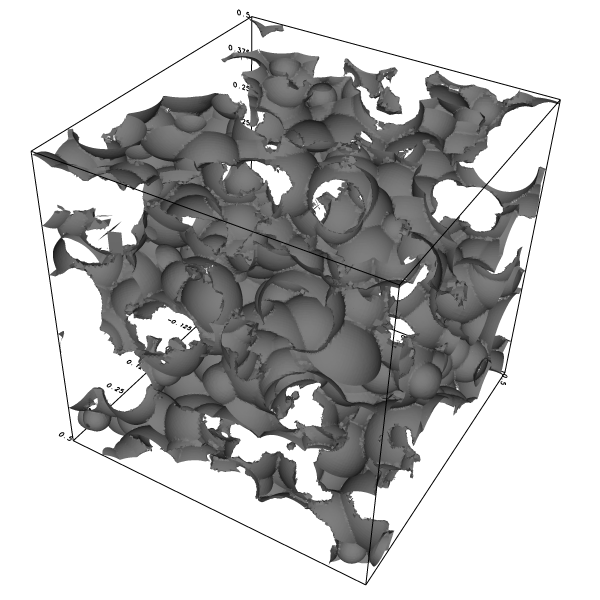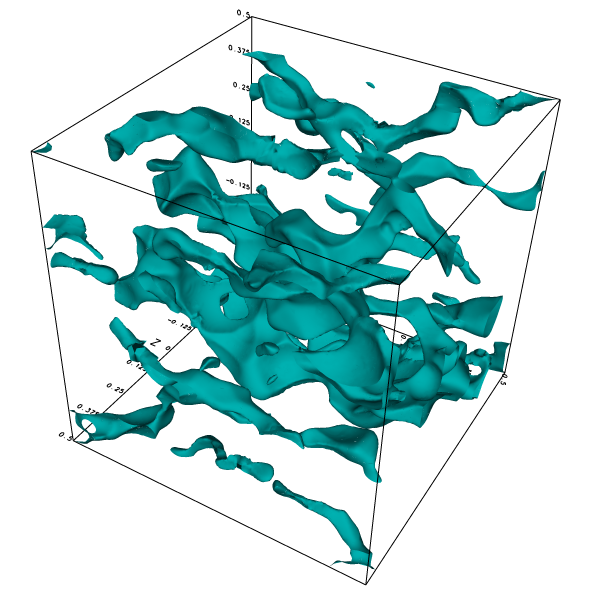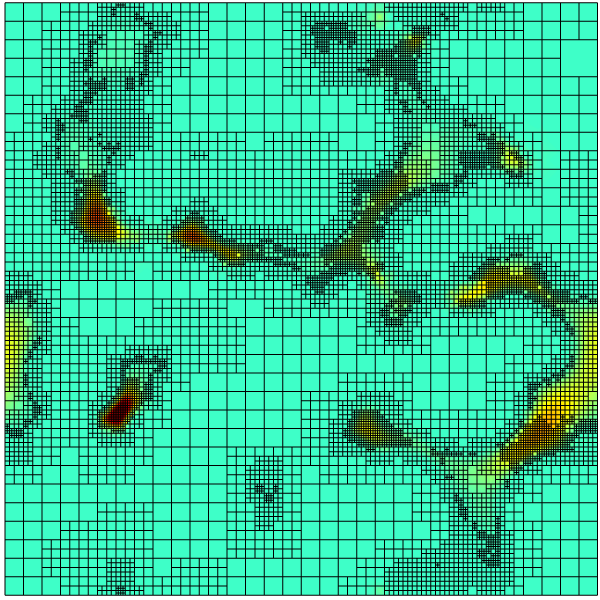# Stokes flow through a complex 3D porous medium

This is the 3D equivalent of the 2D porous medium test case.

The medium is periodic and described using embedded boundaries.

This tests mainly the robustness of the representation of embedded boundaries and the convergence of the viscous and Poisson solvers.

#include "grid/octree.h"
#include "embed.h"
#include "navier-stokes/centered.h"
#include "view.h"
#include "navier-stokes/perfs.h"

We will vary the maximum level of refinement, starting from 5.

int maxlevel = 5;

The porous medium is defined by the union of a random collection of spheres. The number of spheres ns can be varied to vary the porosity.

void porous (scalar cs, face vector fs)
{
int ns = 700;
coord pc[ns];
double R[ns];
srand (0);
for (int i = 0; i < ns; i++) {
foreach_dimension()
pc[i].x = 0.5*noise();
R[i] = 0.04 + 0.08*fabs(noise());
}

Once we have defined the random centers and radii, we can compute the levelset function \phi representing the embedded boundary.

  vertex scalar phi[];
foreach_vertex() {
phi[] = HUGE;

Since the medium is periodic, we need to take into account all the disk images using periodic symmetries. Note that this means that each function evaluation requires 27 times ns evaluations of the function for a sphere. This is expensive but could be improved a lot using a more clever algorithm.

    for (double xp = -L0; xp <= L0; xp += L0)
for (double yp = -L0; yp <= L0; yp += L0)
for (double zp = -L0; zp <= L0; zp += L0)
for (int i = 0; i < ns; i++)
phi[] = intersection (phi[], (sq(x + xp - pc[i].x) +
sq(y + yp - pc[i].y) +
sq(z + zp - pc[i].z) -
sq(R[i])));
}

fractions (phi, cs, fs);

This is necessary to remove degenerate fractions which could cause convergence problems.

  fractions_cleanup (cs, fs);
}

The domain is the periodic unit cube centered on the origin.

int main()
{
origin (-0.5, -0.5, -0.5);
foreach_dimension()
periodic (right);

We turn off the advection term. The choice of the maximum timestep and of the tolerance on the Poisson and viscous solves is not trivial. This was adjusted by trial and error to minimize (possibly) splitting errors and optimize convergence speed.

  stokes = true;
DT = 2e-5;
TOLERANCE = HUGE;
NITERMIN = 2;
N = 1 << maxlevel;

run();
}

scalar un[];

event init (t = 0) {

We define the porous embedded geometry.

  porous (cs, fs);

The gravity vector is aligned with the channel and viscosity is unity.

  const face vector g[] = {1.,0.,0.};
a = g;
mu = fm;

The boundary condition is zero velocity on the embedded boundary.

  u.n[embed] = dirichlet(0);
u.t[embed] = dirichlet(0);
u.r[embed] = dirichlet(0);

We initialize the reference velocity.

  foreach()
un[] = u.x[];
}

#if 0 // used for debugging only
coord maxpos (scalar s)
{
coord pmax = {0,0,0};
double numax = 0.;
foreach (serial)
if (fabs(s[]) > numax) {
numax = fabs(s[]);
pmax = (coord){x,y,z};
}
return pmax;
}

event dumps (i += 10) {
scalar nu[], du[], crappy[];
foreach() {
nu[] = norm(u);
du[] = u.x[] - un[];
double val;
crappy[] = (embed_flux (point, u.x, mu, &val) != 0.);
}

#if !_MPI
coord numax = maxpos (nu);
fprintf (stderr, "numax: %g %g %g\n", numax.x, numax.y, numax.z);
numax = maxpos (p);
#if 0
Point point = locate (numax.x, numax.y, numax.z);
fprintf (stderr, "pmax: %g %g %g %g %g\n", numax.x, numax.y, numax.z,
p[], cs[]);
#endif
numax = maxpos (du);
{
Point point = locate (numax.x, numax.y, numax.z);
fprintf (stderr, "dumax: %g %g %g\n", numax.x, numax.y, numax.z);
FILE * fp = fopen ("dumax", "w");
foreach_neighbor(1) {
fprintf (fp, "fs %g %g %g %g\n", x - Delta/2., y, z, fs.x[]);
fprintf (fp, "fs %g %g %g %g\n", x, y - Delta/2., z, fs.y[]);
fprintf (fp, "fs %g %g %g %g\n", x, y, z - Delta/2., fs.z[]);
fprintf (fp, "fs %g %g %g %g\n", x + Delta/2., y, z, fs.x);
fprintf (fp, "fs %g %g %g %g\n", x, y + Delta/2., z, fs.y[0,1]);
fprintf (fp, "fs %g %g %g %g\n", x, y, z + Delta/2., fs.z[0,0,1]);
fprintf (fp, "%g %g %g %g\n", x, y, z, cs[]);
}
fclose (fp);
}
#endif

p.nodump = false;
dump();
}
#endif

We check for a stationary solution.

event logfile (i++; i <= 500)
{
double avg = normf(u.x).avg, du = change (u.x, un)/(avg + SEPS);
fprintf (stderr, "%d %d %d %d %d %d %d %d %.3g %.3g %.3g %.3g %.3g\n",
maxlevel, i,
mgp.i, mgp.nrelax, mgp.minlevel,
mgu.i, mgu.nrelax, mgu.minlevel,
du, mgp.resa*dt, mgu.resa, statsf(u.x).sum, normf(p).max);

If the relative change of the velocity is small enough we stop this simulation.

  if (i > 1 && (avg < 1e-9 || du < 0.01)) {

We are interested in the permeability k of the medium, which is defined by \displaystyle U = \frac{k}{\mu}\nabla p = \frac{k}{\mu}\rho g with U the average fluid velocity.

    stats s = statsf (u.x);
printf ("%d %g\n", maxlevel, s.sum/s.volume);

We output fields and dump the simulation.

    scalar nu[];
foreach()
nu[] = norm(u);

view (fov = 32.2073, quat = {-0.309062,0.243301,0.0992085,0.914026},
tx = 0.0122768, ty = 0.0604286, bg = {1,1,1},
width = 600, height = 600);
box();
draw_vof("cs", "fs", fc = {0.5,0.5,0.5});
char name;
sprintf (name, "cs-%d.png", maxlevel);
save (name);

box();
isosurface ("u.x", 1e-5, fc = {0.,0.7,0.7});
sprintf (name, "nu-%d.png", maxlevel);
save (name);

view (fov = 19.1765, quat = {0,0,0,1},
tx = 0.0017678, ty = 0.00157844, bg = {1,1,1},
width = 600, height = 600);
squares ("nu", linear = true);
cells();
sprintf (name, "cross-%d.png", maxlevel);
save (name);

sprintf (name, "dump-%d", maxlevel);
dump (name);

We stop at level 8.

    if (maxlevel >= 8)
return 1; /* stop */

We refine the converged solution to get the initial guess for the finer level. We also reset the embedded fractions to avoid interpolation errors on the geometry.

    maxlevel++;
#if TREE
#endif
porous (cs, fs);
}
}Boundary of the porous medium. Fluid is flowing inside this volume.Isosurface of the x-component of the velocity.Cross-section at z = 0 showing the mesh and norm of the velocity.

set xlabel 'Level'
set grid
set ytics format '%.1e'
plot 'out' w lp t ''Permeability as a function of resolution (script)

set xlabel 'Iterations'
set logscale y
set ytics format '%.0e'
set yrange [1e-8:]
set key center left
plot '../porous3D.ref' u 2:9 w l t '', '' u 2:10 w l t '', \
'' u 2:11 w l t '', '' u 2:12 w l t '', '' u 2:13 w l t '', \
'log' u 2:9 w p t 'du', '' u 2:10 w p t 'resp', \
'' u 2:11 w p t 'resu', '' u 2:12 w p t 'u.x.sum', '' u 2:13 w p t 'p.max'Convergence history (script)